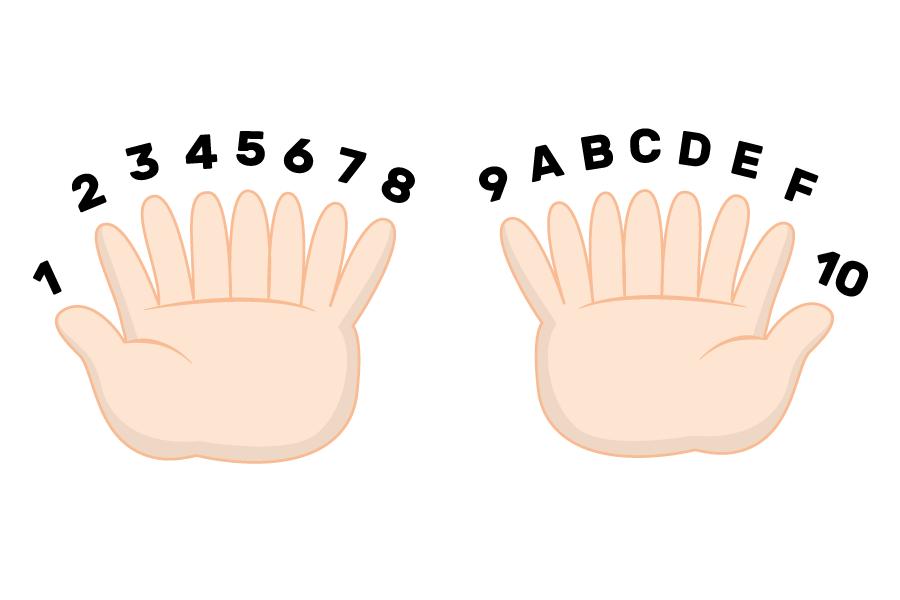## Want to keep learning?

This content is taken from the Raspberry Pi Foundation & National Centre for Computing Education's online course, Understanding Maths and Logic in Computer Science. Join the course to learn more.
3.7

# An Introduction to Hexadecimal Numbers

Although numbers are processed inside a computer as binary, that doesn’t mean that we need to always represent these numbers as binary to humans.

Which of the following numbers is easier to remember?

• 1010 0101
• A5

If you think A5 is easier and more convenient to remember and use, you now understand why some binary is expressed in hexadecimal format. A5 is actually the same value as 1010 0101; however, it is represented as hexadecimal instead of binary.

We often see hexadecimal used to represent binary numbers for:

• Colours. These are often represented as intensities of red, green, and blue light, each of which can be represented by two hexadecimal digits. See our Representing Data course for more.
• MAC addresses used in networking. See our Introduction to Computer Networking course for more.
• Memory addresses: the specific place in memory in which a piece of data is stored. These are often important in error messages.

Hexadecimal is a base 16 number system: it makes use of 16 numbers. So if we had a creature with 16 fingers, this is how they would count using hexadecimal:This table shows equivalent denary, binary, and hexadecimal values:

Denary Binary Hex
0 0000 0
1 0001 1
2 0010 2
3 0011 3
4 0100 4
5 0101 5
6 0110 6
7 0111 7
8 1000 8
9 1001 9
10 1010 A
11 1011 B
12 1100 C
13 1101 D
14 1110 E
15 1111 F

Hexadecimal is commonly used in computing because it can represent a byte of data with just two characters (instead of eight). Each hexadecimal character represents half a byte, also called a nibble.

• So, hexadecimal 7D is equivalent to binary 0111 1101.

Simiarly, the binary number 0001 1010 is converted to hexadecimal like this:

• 0001 is the binary representation of 1 (which is the same in denary and hexadecimal).
• 1010 is the binary representation of the denary number 10, which is represented in hexadecimal as A.
• So, binary 0001 1010 is equivalent to hexadecimal 1A.

### Converting hexadecimal to denary, via binary

You already know how to convert binary numbers to denary, and since you just learned how to convert a hexadecimal number to binary, you can also convert it to denary.

For example, the number A9 in hexadecimal is 1010 1001 in binary. And we can convert this to denary using the table we’ve used before:

128 64 32 16 8 4 2 1
1 0 1 0 1 0 0 1

128 + 32 + 8 + 1 = 169

### Converting hexadecimal directly to denary

We can also convert hexadecimal directly into denary by thinking about the place values. You know that the place values of denary numbers work like this:

1089 = (1 × 1000) + (0 × 100) + (8 × 10) + (9 × 1)

Base 10 place values 1000 (10³) 100 (10²) 10 (10¹) 1 (10⁰)
Denary value 1 0 8 9

Hexadecimal works very similarly, with base 16 instead of base 10. Let’s convert hexadecimal A9 to denary:

Base 16 place values 16 (16¹) 1 (16⁰)
• From the table above, we know that hexadecimal A represents denary 10 and hexadecimal 9 represents denary 9.
• Just like in 1089, there is a 9 in the 1 position: (9 × 1).
• There is a 10 in the 16 position: (10 × 16).
• So that means hexadecimal A9 = (10 × 16) + (9 × 1) = 169 in denary.
Base 16 place values 16 (16¹) 1 (16⁰)
Conversion (10 × 16) = 160 (9 × 1) = 9

Let’s convert denary 200 to hexadecimal. We do this by finding out how many times 16 fits into 200 in denary in order to represent 200 in base 16:

• 200 / 16 = 12, remainder 8.
• So, we have 12 lots of 16, with 8 units left over.
• Denary 12 is hexadecimal C, and denary 8 is hexadecimal 8 (check out the table above if you forget).
• So, denary 200 = (12 × 16) + (8 × 1) = C8 in hexadecimal.